# Ichigo: The Agricultural Bot

Ichigo is an agricultural robot that goes around the cultvational land and gets you statistics about the diseased plants and the field.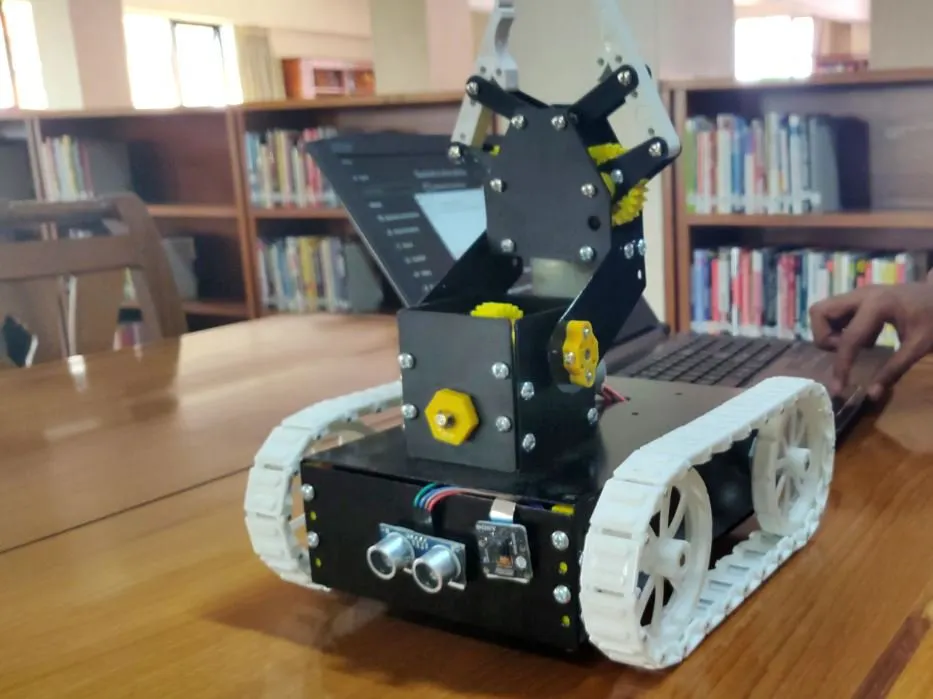## Things used in this project

### Hardware components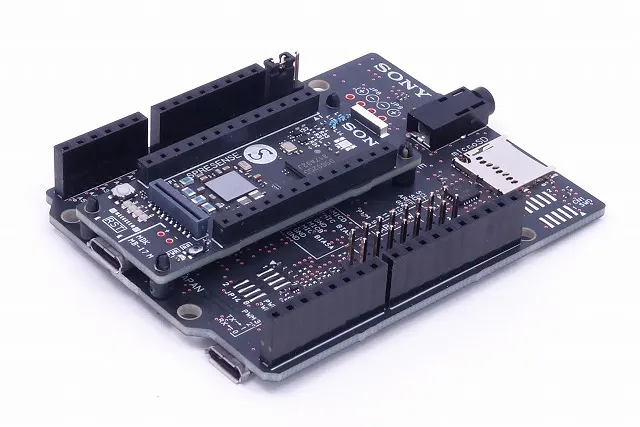Sony Spresense boards (main & extension)
×1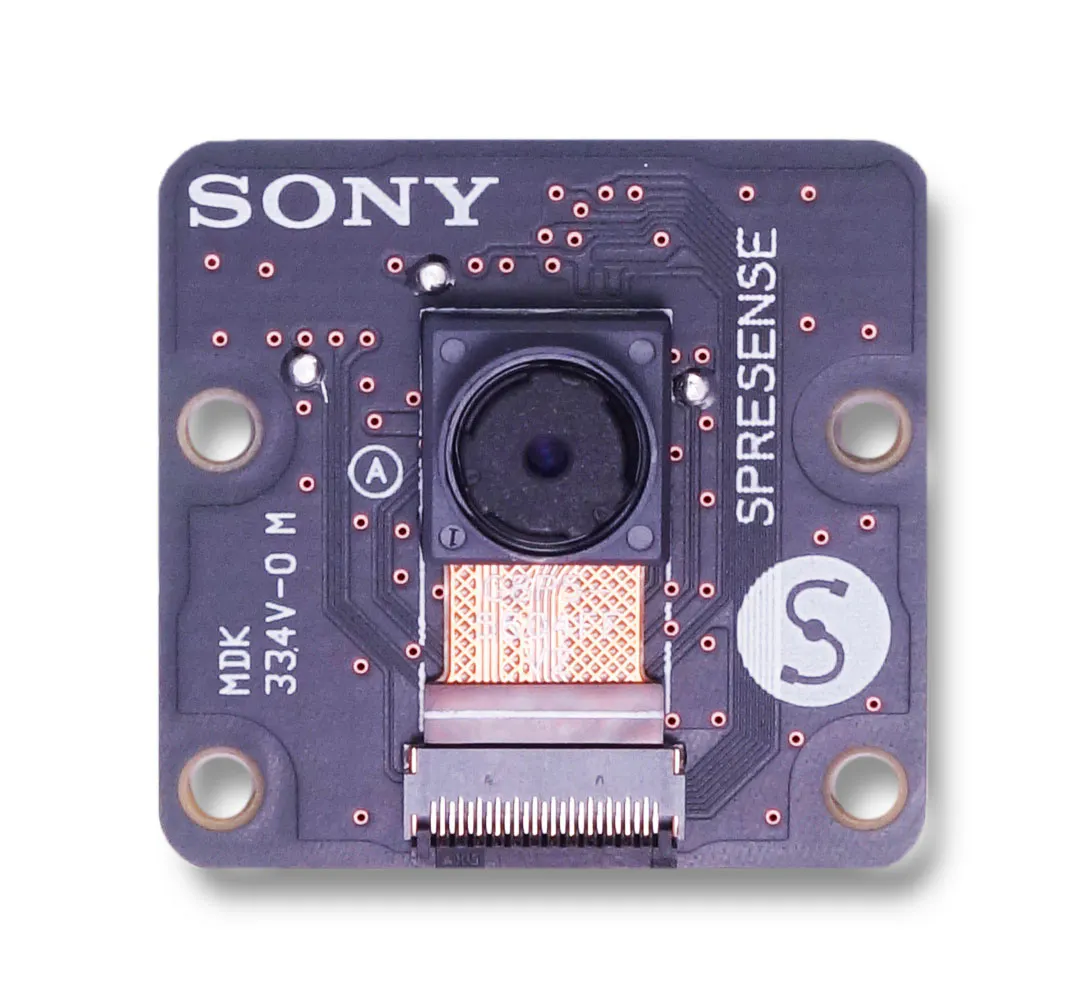Sony Spresense camera board
×1Ultrasonic Sensor - HC-SR04 (Generic)
×1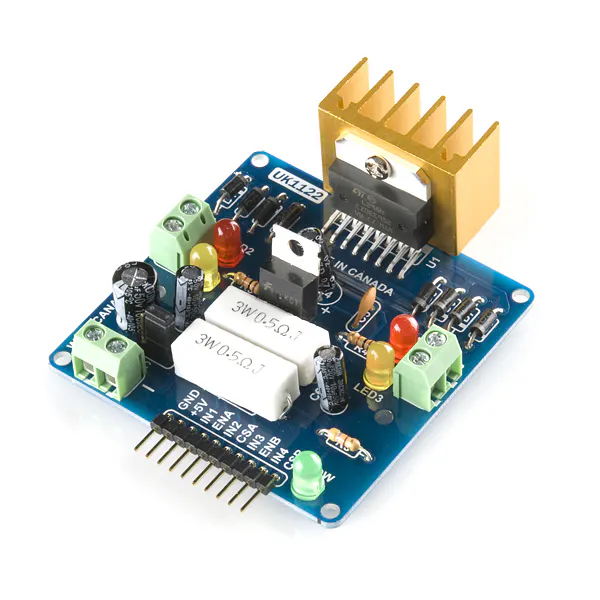SparkFun Dual H-Bridge motor drivers L298
×2

### Software apps and online servicesArduino IDE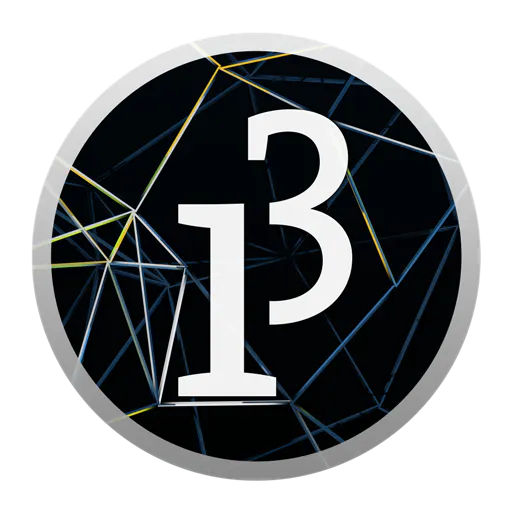The Processing Foundation Processing
 DS4 tools for windows

## Schematics

### Connections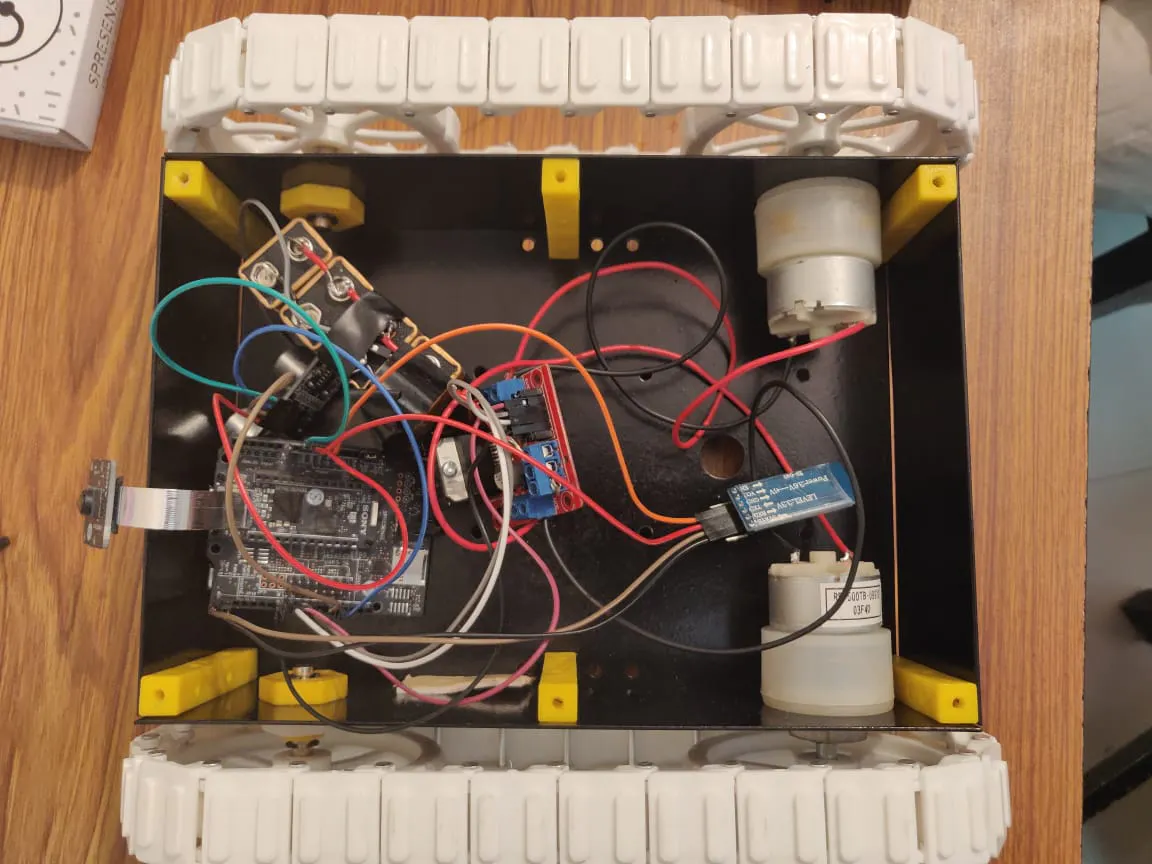## Code

### Processing code

C/C++
This is the processing code to send the values to the bluetooth module of arduino
```import processing.serial.*;

Serial myPort;  // Create object from Serial class

void setup()
{
size(200,200); //make our canvas 200 x 200 pixels big
println(Serial.list());
String portName = Serial.list(); //change the 0 to a 1 or 2 etc. to match your port
myPort = new Serial(this, portName, 9600);
}
void draw()
{

int count=0;
if (keyPressed == true)
{
if (key=='d')
{ myPort.write("d");
println(key);
delay(100);
}
if (key=='s')
{ myPort.write("s");
println(key);
delay(100);
}
if (key=='a')
{ myPort.write("a");
println(key);
delay(100);
}
if (key=='w')
{ myPort.write("w");
println(key);
delay(100);
}
if (key=='i')
{ myPort.write("i");
println(key);
delay(100);
}
if (key=='k')
{ myPort.write("k");
println(key);
delay(100);
}
if (key=='j')
{ myPort.write("j");
println(key);
delay(100);
}
if (key=='l')
{ myPort.write("l");
println(key);
delay(100);
}
if (key=='c')
{ myPort.write("c");
println(key);
delay(100);
}
}
else
println("__INVALID/NO INPUT__");
}
```

### Sony Board Code - Camera, GPS, and Ultrasonic Sensor

C/C++
This is the code that runs on the robot and includes code for the camera, bluetooth, ultrasonic sensor and the GPS
```#include <SDHCI.h>
#include <stdio.h>  /* for sprintf */

#include <Camera.h>
#include <GNSS.h>

#define STRING_BUFFER_SIZE  128       /**< %Buffer size */

#define RESTART_CYCLE       (60 * 5)  /**< positioning test term */

static SpGnss Gnss;                   /**< SpGnss object */

#define BAUDRATE                (9600)

/**
* @enum ParamSat
* @brief Satellite system
*/
enum ParamSat {
eSatGps,            /**< GPS                     World wide coverage  */
eSatGlonass,        /**< GLONASS                 World wide coverage  */
eSatGpsSbas,        /**< GPS+SBAS                North America        */
eSatGpsGlonass,     /**< GPS+Glonass             World wide coverage  */
eSatGpsQz1c,        /**< GPS+QZSS_L1CA           East Asia & Oceania  */
eSatGpsGlonassQz1c, /**< GPS+Glonass+QZSS_L1CA   East Asia & Oceania  */
eSatGpsQz1cQz1S,    /**< GPS+QZSS_L1CA+QZSS_L1S  Japan                */
};

/* Set this parameter depending on your current region. */
static enum ParamSat satType =  eSatGps;

/**
* @brief Turn on / off the LED0 for CPU active notification.
*/
static void Led_isActive(void)
{
static int state = 1;
if (state == 1)
{
ledOn(PIN_LED0);
state = 0;
}
else
{
ledOff(PIN_LED0);
state = 1;
}
}

/**
* @brief Turn on / off the LED1 for positioning state notification.
*
* @param [in] state Positioning state
*/
static void Led_isPosfix(bool state)
{
if (state)
{
ledOn(PIN_LED1);
}
else
{
ledOff(PIN_LED1);
}
}

/**
* @brief Turn on / off the LED3 for error notification.
*
* @param [in] state Error state
*/
static void Led_isError(bool state)
{
if (state)
{
ledOn(PIN_LED3);
}
else
{
ledOff(PIN_LED3);
}
}

/**
* @brief Activate GNSS device and start positioning.
*/
SDClass  theSD;
int take_picture_count = 0;

/**
* Callback from Camera library when video frame is captured.
*/

void CamCB(CamImage img)
{

/* Check the img instance is available or not. */

if (img.isAvailable())
{

/* If you want RGB565 data, convert image data format to RGB565 */

img.convertPixFormat(CAM_IMAGE_PIX_FMT_RGB565);

/* You can use image data directly by using getImgSize() and getImgBuff().
* for displaying image to a display, etc. */

Serial.print("Image data size = ");
Serial.print(img.getImgSize(), DEC);
Serial.print(" , ");

Serial.print((unsigned long)img.getImgBuff(), HEX);
Serial.println("");
}
else
{
Serial.print("Failed to get video stream image\n");
}
}

/**
* @brief Initialize camera
*/
//Motor A left motor
const int motorPin1  = 9;  // Pin 14 of L293
const int motorPin2  = 10;  // Pin 10 of L293
//Motor B right motor
const int motorPin3  = 8; // Pin  7 of L293
const int motorPin4  = 7;  // Pin  2 of L293
//Arm Motor 1
const int motorPin5  = 5;
const int motorPin6  = 6;
//Arm Motor 2
const int motorPin7  = 3;
const int motorPin8  = 4;
char val;
// defines pins numbers for ultrasonic sensor
const int trigPin = 12;
const int echoPin = 13;
// defines variables for ultrasonic sensor
long duration;
int distance;
void setup() {

/* put your setup code here, to run once: */

int error_flag = 0;

/* Wait HW initialization done. */
sleep(3);

/* Turn on all LED:Setup start. */
ledOn(PIN_LED0);
ledOn(PIN_LED1);
ledOn(PIN_LED2);
ledOn(PIN_LED3);

/* Set Debug mode to Info */
Gnss.setDebugMode(PrintInfo);

int result;

/* Activate GNSS device */
result = Gnss.begin();

if (result != 0)
{
Serial.println("Gnss begin error!!");
error_flag = 1;
}
else
{
/* Setup GNSS
*  It is possible to setup up to two GNSS satellites systems.
*  Depending on your location you can improve your accuracy by selecting different GNSS system than the GPS system.
*  See: https://developer.sony.com/develop/spresense/developer-tools/get-started-using-nuttx/nuttx-developer-guide#_gnss
*  for detailed information.
*/
switch (satType)
{
case eSatGps:
Gnss.select(GPS);
break;

case eSatGpsSbas:
Gnss.select(GPS);
Gnss.select(SBAS);
break;

case eSatGlonass:
Gnss.select(GLONASS);
break;

case eSatGpsGlonass:
Gnss.select(GPS);
Gnss.select(GLONASS);
break;

case eSatGpsQz1c:
Gnss.select(GPS);
Gnss.select(QZ_L1CA);
break;

case eSatGpsQz1cQz1S:
Gnss.select(GPS);
Gnss.select(QZ_L1CA);
Gnss.select(QZ_L1S);
break;

case eSatGpsGlonassQz1c:
default:
Gnss.select(GPS);
Gnss.select(GLONASS);
Gnss.select(QZ_L1CA);
break;
}

/* Start positioning */
result = Gnss.start(COLD_START);
if (result != 0)
{
Serial.println("Gnss start error!!");
error_flag = 1;
}
else
{
Serial.println("Gnss setup OK");
}
}

/* Turn off all LED:Setup done. */
ledOff(PIN_LED0);
ledOff(PIN_LED1);
ledOff(PIN_LED2);
ledOff(PIN_LED3);

/* Set error LED. */
if (error_flag == 1)
{
Led_isError(true);
exit(0);
}
/* Open serial communications and wait for port to open */

Serial.begin(BAUDRATE);
while (!Serial)
{
; /* wait for serial port to connect. Needed for native USB port only */
}

/* begin() without parameters means that
* number of buffers = 1, 30FPS, QVGA, YUV 4:2:2 format */

Serial.println("Prepare camera");
theCamera.begin();

/* Start video stream.
* If received video stream data from camera device,
*  camera library call CamCB.
*/

Serial.println("Start streaming");
theCamera.startStreaming(true, CamCB);

/* Auto white balance configuration */

Serial.println("Set Auto white balance parameter");
theCamera.setAutoWhiteBalanceMode(CAM_WHITE_BALANCE_DAYLIGHT);

/* Set parameters about still picture.
* In the following case, QUADVGA and JPEG.
*/

Serial.println("Start streaming");
theCamera.setStillPictureImageFormat(
CAM_IMAGE_PIX_FMT_JPG);
pinMode(motorPin1, OUTPUT);
pinMode(motorPin2, OUTPUT);
pinMode(motorPin3, OUTPUT);
pinMode(motorPin4, OUTPUT);
pinMode(motorPin5, OUTPUT);
pinMode(motorPin6, OUTPUT);
pinMode(motorPin7, OUTPUT);
pinMode(motorPin8, OUTPUT);
pinMode(trigPin, OUTPUT); // Sets the trigPin as an Output
pinMode(echoPin, INPUT); // Sets the echoPin as an Input
// Serial.begin(9600);

}

/**
* @brief %Print position information.
*/
static void print_pos(SpNavData *pNavData)
{
char StringBuffer[STRING_BUFFER_SIZE];

/* print time */
snprintf(StringBuffer, STRING_BUFFER_SIZE, "%04d/%02d/%02d ", pNavData->time.year, pNavData->time.month, pNavData->time.day);
Serial.print(StringBuffer);

snprintf(StringBuffer, STRING_BUFFER_SIZE, "%02d:%02d:%02d.%06d, ", pNavData->time.hour, pNavData->time.minute, pNavData->time.sec, pNavData->time.usec);
Serial.print(StringBuffer);

/* print satellites count */
snprintf(StringBuffer, STRING_BUFFER_SIZE, "numSat:%2d, ", pNavData->numSatellites);
Serial.print(StringBuffer);

/* print position data */
if (pNavData->posFixMode == FixInvalid)
{
Serial.print("No-Fix, ");
}
else
{
Serial.print("Fix, ");
}
if (pNavData->posDataExist == 0)
{
Serial.print("No Position");
}
else
{
Serial.print("Lat=");
Serial.print(pNavData->latitude, 6);
Serial.print(", Lon=");
Serial.print(pNavData->longitude, 6);
}

Serial.println("");
}

/**
* @brief %Print satellite condition.
*/
static void print_condition(SpNavData *pNavData)
{
char StringBuffer[STRING_BUFFER_SIZE];
unsigned long cnt;

/* Print satellite count. */
snprintf(StringBuffer, STRING_BUFFER_SIZE, "numSatellites:%2d\n", pNavData->numSatellites);
Serial.print(StringBuffer);

for (cnt = 0; cnt < pNavData->numSatellites; cnt++)
{
const char *pType = "---";
SpSatelliteType sattype = pNavData->getSatelliteType(cnt);

/* Get satellite type. */
/* Keep it to three letters. */
switch (sattype)
{
case GPS:
pType = "GPS";
break;

case GLONASS:
pType = "GLN";
break;

case QZ_L1CA:
pType = "QCA";
break;

case SBAS:
pType = "SBA";
break;

case QZ_L1S:
pType = "Q1S";
break;

default:
pType = "UKN";
break;
}

/* Get print conditions. */
unsigned long Id  = pNavData->getSatelliteId(cnt);
unsigned long Elv = pNavData->getSatelliteElevation(cnt);
unsigned long Azm = pNavData->getSatelliteAzimuth(cnt);
float sigLevel = pNavData->getSatelliteSignalLevel(cnt);

/* Print satellite condition. */
snprintf(StringBuffer, STRING_BUFFER_SIZE, "[%2d] Type:%s, Id:%2d, Elv:%2d, Azm:%3d, CN0:", cnt, pType, Id, Elv, Azm );
Serial.print(StringBuffer);
Serial.println(sigLevel, 6);
}
}

/**
* @brief %Print position information and satellite condition.
*
* @details When the loop count reaches the RESTART_CYCLE value, GNSS device is
*          restarted.
*/

void loop() {
static int LoopCount = 0;
static int LastPrintMin = 0;

Led_isActive();

/* Check update. */
if (Gnss.waitUpdate(-1))
{
/* Get NaviData. */
SpNavData NavData;
Gnss.getNavData(&NavData);

/* Set posfix LED. */
bool LedSet = (NavData.posDataExist && (NavData.posFixMode != FixInvalid));
Led_isPosfix(LedSet);

/* Print satellite information every minute. */
if (NavData.time.minute != LastPrintMin)
{
print_condition(&NavData);
LastPrintMin = NavData.time.minute;
}

/* Print position information. */
print_pos(&NavData);
}
else
{
/* Not update. */
Serial.println("data not update");
}

/* Check loop count. */
LoopCount++;
if (LoopCount >= RESTART_CYCLE)
{
int error_flag = 0;

/* Turn off LED0 */
ledOff(PIN_LED0);

/* Set posfix LED. */
Led_isPosfix(false);

/* Restart GNSS. */
if (Gnss.stop() != 0)
{
Serial.println("Gnss stop error!!");
error_flag = 1;
}
else if (Gnss.end() != 0)
{
Serial.println("Gnss end error!!");
error_flag = 1;
}
else
{
Serial.println("Gnss stop OK.");
}

if (Gnss.begin() != 0)
{
Serial.println("Gnss begin error!!");
error_flag = 1;
}
else if (Gnss.start(HOT_START) != 0)
{
Serial.println("Gnss start error!!");
error_flag = 1;
}
else
{
Serial.println("Gnss restart OK.");
}

LoopCount = 0;

/* Set error LED. */
if (error_flag == 1)
{
Led_isError(true);
exit(0);
}
}
digitalWrite(trigPin, LOW);
delayMicroseconds(2);
// Sets the trigPin on HIGH state for 10 micro seconds
digitalWrite(trigPin, HIGH);
delayMicroseconds(10);
digitalWrite(trigPin, LOW);
// Reads the echoPin, returns the sound wave travel time in microseconds
duration = pulseIn(echoPin, HIGH);
// Calculating the distance
distance= duration*0.034/2;
delay(500);
// Prints the distance on the Serial Monitor
Serial.print("Distance: ");
Serial.println(distance);
if (Serial.available())
{
delay(100);
if (val == 's')
{
//Backward code
// Set Motor A backward
digitalWrite(motorPin1, HIGH);
digitalWrite(motorPin2, LOW);
// Set Motor B backward
digitalWrite(motorPin3, HIGH);
digitalWrite(motorPin4, LOW);
Serial.println("Backward");

}
if (val == 'w')
{
//forward code
// Set Motor A forward
digitalWrite(motorPin1, LOW);
digitalWrite(motorPin2, HIGH);
// Set Motor B forward
digitalWrite(motorPin3, LOW);
digitalWrite(motorPin4, HIGH);
//  delay(2000);
}
if (val == 'a')
{
//left code
// Set Motor A backward
digitalWrite(motorPin1, HIGH);
digitalWrite(motorPin2, LOW);
// Set Motor B forward
digitalWrite(motorPin3, LOW);
digitalWrite(motorPin4, HIGH);
//delay(2000);
}
if (val == 'd')
{
//right code
// Set Motor A forward
digitalWrite(motorPin1, LOW);
digitalWrite(motorPin2, HIGH);
// Set Motor B backward
digitalWrite(motorPin3, HIGH);
digitalWrite(motorPin4, LOW);
//delay(2000);
}
if (val == 'i')
{
//Arm motor 1 operation 1
digitalWrite(motorPin5, LOW);
digitalWrite(motorPin6, HIGH);
}
if (val == 'k')
{
//Arm motor 1 operation 2
digitalWrite(motorPin5, HIGH);
digitalWrite(motorPin6, LOW);
}
if (val == 'j')
{
//Arm motor 2 operation 1
digitalWrite(motorPin7, HIGH);
digitalWrite(motorPin8, LOW);
}
if (val == 'l')
{
//Arm motor 2 operation 2
digitalWrite(motorPin7, LOW);
digitalWrite(motorPin8, HIGH);
}
if (val == 'c')
{
sleep(1); /* wait for one second to take still picture. */

/* You can change the format of still picture at here also, if you want. */

/* theCamera.setStillPictureImageFormat(
*   CAM_IMGSIZE_HD_H,
*   CAM_IMGSIZE_HD_V,
*   CAM_IMAGE_PIX_FMT_JPG);
*/

/* This sample code can take 100 pictures in every one second from starting. */

if (take_picture_count < 100)
{

/* Take still picture.
* Unlike video stream(startStreaming) , this API wait to receive image data
*  from camera device.
*/

Serial.println("call takePicture()");
CamImage img = theCamera.takePicture();

/* Check availability of the img instance. */
/* If any error was occured, the img is not available. */

if (img.isAvailable())
{
/* Create file name */

char filename = {0};
sprintf(filename, "PICT%03d.JPG", take_picture_count);

Serial.print("Save taken picture as ");
Serial.print(filename);
Serial.println("");

/* Save to SD card as the finename */

File myFile = theSD.open(filename, FILE_WRITE);
myFile.write(img.getImgBuff(), img.getImgSize());
myFile.close();
}

take_picture_count++;
}
}
}
else
{
Serial.println("Serial not available");
digitalWrite(motorPin1, LOW);
digitalWrite(motorPin2, LOW);
digitalWrite(motorPin3, LOW);
digitalWrite(motorPin4, LOW);
digitalWrite(motorPin5, LOW);
digitalWrite(motorPin6, LOW);
digitalWrite(motorPin7, LOW);
digitalWrite(motorPin8, LOW);
}
}
```

### Image Processing Code

Python
```# Copyright (c) 2017 Sony Corporation. All Rights Reserved.
#
# you may not use this file except in compliance with the License.
# You may obtain a copy of the License at
#
#
# Unless required by applicable law or agreed to in writing, software
# WITHOUT WARRANTIES OR CONDITIONS OF ANY KIND, either express or implied.
# See the License for the specific language governing permissions and

import nnabla as nn
import nnabla.logger as logger
import nnabla.functions as F
import nnabla.parametric_functions as PF
import nnabla.solver as S
import nnabla.utils.save as save

from args import get_args
from mnist_data import data_iterator_mnist

import os

def categorical_error(pred, label):
"""
Compute categorical error given score vectors and labels as
numpy.ndarray.
"""
pred_label = pred.argmax(1)
return (pred_label != label.flat).mean()

# Binary Connect Model
def mnist_binary_connect_lenet_prediction(image, test=False):
"""
Construct LeNet for MNIST (BinaryNet version).
"""
with nn.parameter_scope("conv1"):
c1 = PF.binary_connect_convolution(image, 16, (5, 5))
c1 = PF.batch_normalization(c1, batch_stat=not test)
c1 = F.elu(F.average_pooling(c1, (2, 2)))
with nn.parameter_scope("conv2"):
c2 = PF.binary_connect_convolution(c1, 16, (5, 5))
c2 = PF.batch_normalization(c2, batch_stat=not test)
c2 = F.elu(F.average_pooling(c2, (2, 2)))
with nn.parameter_scope("fc3"):
c3 = PF.binary_connect_affine(c2, 50)
c3 = PF.batch_normalization(c3, batch_stat=not test)
c3 = F.elu(c3)
with nn.parameter_scope("fc4"):
c4 = PF.binary_connect_affine(c3, 10)
c4 = PF.batch_normalization(c4, batch_stat=not test)
return c4

def mnist_binary_connect_resnet_prediction(image, test=False):
"""
Construct ResNet for MNIST (BinaryNet version).
"""
def bn(x):
return PF.batch_normalization(x, batch_stat=not test)

def res_unit(x, scope):
C = x.shape
with nn.parameter_scope(scope):
with nn.parameter_scope('conv1'):
h = F.elu(bn(PF.binary_connect_convolution(
x, C / 2, (1, 1), with_bias=False)))
with nn.parameter_scope('conv2'):
h = F.elu(
bn(PF.binary_connect_convolution(h, C / 2, (3, 3), pad=(1, 1), with_bias=False)))
with nn.parameter_scope('conv3'):
h = bn(PF.binary_connect_convolution(
h, C, (1, 1), with_bias=False))
return F.elu(x + h)
# Conv1 --> 64 x 32 x 32
with nn.parameter_scope("conv1"):
c1 = F.elu(
bn(PF.binary_connect_convolution(image, 64, (3, 3), pad=(3, 3), with_bias=False)))
# Conv2 --> 64 x 16 x 16
c2 = F.max_pooling(res_unit(c1, "conv2"), (2, 2))
# Conv3 --> 64 x 8 x 8
c3 = F.max_pooling(res_unit(c2, "conv3"), (2, 2))
# Conv4 --> 64 x 8 x 8
c4 = res_unit(c3, "conv4")
# Conv5 --> 64 x 4 x 4
c5 = F.max_pooling(res_unit(c4, "conv5"), (2, 2))
# Conv5 --> 64 x 4 x 4
c6 = res_unit(c5, "conv6")
pl = F.average_pooling(c6, (4, 4))
with nn.parameter_scope("classifier"):
y = bn(PF.binary_connect_affine(pl, 10))
return y

# Binary Net Model

def mnist_binary_net_lenet_prediction(image, test=False):
"""
Construct LeNet for MNIST (BinaryNet version).
"""
with nn.parameter_scope("conv1"):
c1 = PF.binary_connect_convolution(image, 16, (5, 5))
c1 = PF.batch_normalization(c1, batch_stat=not test)
c1 = F.binary_tanh(F.average_pooling(c1, (2, 2)))
with nn.parameter_scope("conv2"):
c2 = PF.binary_connect_convolution(c1, 16, (5, 5))
c2 = PF.batch_normalization(c2, batch_stat=not test)
c2 = F.binary_tanh(F.average_pooling(c2, (2, 2)))
with nn.parameter_scope("fc3"):
c3 = PF.binary_connect_affine(c2, 50)
c3 = PF.batch_normalization(c3, batch_stat=not test)
c3 = F.binary_tanh(c3)
with nn.parameter_scope("fc4"):
c4 = PF.binary_connect_affine(c3, 10)
c4 = PF.batch_normalization(c4, batch_stat=not test)
return c4

def mnist_binary_net_resnet_prediction(image, test=False):
"""
Construct ResNet for MNIST (BinaryNet version).
"""
def bn(x):
return PF.batch_normalization(x, batch_stat=not test)

def res_unit(x, scope):
C = x.shape
with nn.parameter_scope(scope):
with nn.parameter_scope('conv1'):
h = F.binary_tanh(bn(PF.binary_connect_convolution(
x, C / 2, (1, 1), with_bias=False)))
with nn.parameter_scope('conv2'):
h = F.binary_tanh(
bn(PF.binary_connect_convolution(h, C / 2, (3, 3), pad=(1, 1), with_bias=False)))
with nn.parameter_scope('conv3'):
h = bn(PF.binary_connect_convolution(
h, C, (1, 1), with_bias=False))
return F.binary_tanh(x + h)
# Conv1 --> 64 x 32 x 32
with nn.parameter_scope("conv1"):
c1 = F.binary_tanh(
bn(PF.binary_connect_convolution(image, 64, (3, 3), pad=(3, 3), with_bias=False)))
# Conv2 --> 64 x 16 x 16
c2 = F.max_pooling(res_unit(c1, "conv2"), (2, 2))
# Conv3 --> 64 x 8 x 8
c3 = F.max_pooling(res_unit(c2, "conv3"), (2, 2))
# Conv4 --> 64 x 8 x 8
c4 = res_unit(c3, "conv4")
# Conv5 --> 64 x 4 x 4
c5 = F.max_pooling(res_unit(c4, "conv5"), (2, 2))
# Conv5 --> 64 x 4 x 4
c6 = res_unit(c5, "conv6")
pl = F.average_pooling(c6, (4, 4))
with nn.parameter_scope("classifier"):
y = bn(PF.binary_connect_affine(pl, 10))
return y

# Binary Weight Model

def mnist_binary_weight_lenet_prediction(image, test=False):
"""
Construct LeNet for MNIST (Binary Weight Network version).
"""
with nn.parameter_scope("conv1"):
c1 = PF.binary_weight_convolution(image, 16, (5, 5))
c1 = F.elu(F.average_pooling(c1, (2, 2)))
with nn.parameter_scope("conv2"):
c2 = PF.binary_weight_convolution(c1, 16, (5, 5))
c2 = F.elu(F.average_pooling(c2, (2, 2)))
with nn.parameter_scope("fc3"):
c3 = F.elu(PF.binary_weight_affine(c2, 50))
with nn.parameter_scope("fc4"):
c4 = PF.binary_weight_affine(c3, 10)
return c4

def mnist_binary_weight_resnet_prediction(image, test=False):
"""
Construct ResNet for MNIST (Binary Weight Network version).
"""
def bn(x):
return PF.batch_normalization(x, batch_stat=not test)

def res_unit(x, scope):
C = x.shape
with nn.parameter_scope(scope):
with nn.parameter_scope('conv1'):
h = F.elu(bn(PF.binary_weight_convolution(
x, C / 2, (1, 1), with_bias=False)))
with nn.parameter_scope('conv2'):
h = F.elu(
bn(PF.binary_weight_convolution(h, C / 2, (3, 3), pad=(1, 1), with_bias=False)))
with nn.parameter_scope('conv3'):
h = bn(PF.binary_weight_convolution(
h, C, (1, 1), with_bias=False))
return F.elu(x + h)
# Conv1 --> 64 x 32 x 32
with nn.parameter_scope("conv1"):
c1 = F.elu(
bn(PF.binary_weight_convolution(image, 64, (3, 3), pad=(3, 3), with_bias=False)))
# Conv2 --> 64 x 16 x 16
c2 = F.max_pooling(res_unit(c1, "conv2"), (2, 2))
# Conv3 --> 64 x 8 x 8
c3 = F.max_pooling(res_unit(c2, "conv3"), (2, 2))
# Conv4 --> 64 x 8 x 8
c4 = res_unit(c3, "conv4")
# Conv5 --> 64 x 4 x 4
c5 = F.max_pooling(res_unit(c4, "conv5"), (2, 2))
# Conv5 --> 64 x 4 x 4
c6 = res_unit(c5, "conv6")
pl = F.average_pooling(c6, (4, 4))
with nn.parameter_scope("classifier"):
y = PF.binary_weight_affine(pl, 10)
return y

def train():
"""
Main script.

Steps:

* Parse command line arguments.
* Specify a context for computation.
* Initialize DataIterator for MNIST.
* Construct a computation graph for training and validation.
* Initialize a solver and set parameter variables to it.
* Create monitor instances for saving and displaying training stats.
* Training loop
* Computate error rate for validation data (periodically)
* Get a next minibatch.
* Execute forwardprop on the training graph.
* Execute backprop.
* Compute training error
"""
args = get_args(monitor_path='tmp.monitor.bnn')

# Get context.
from nnabla.ext_utils import get_extension_context
logger.info("Running in %s" % args.context)
ctx = get_extension_context(
args.context, device_id=args.device_id, type_config=args.type_config)
nn.set_default_context(ctx)

# Initialize DataIterator for MNIST.
data = data_iterator_mnist(args.batch_size, True)
vdata = data_iterator_mnist(args.batch_size, False)

# Create CNN network for both training and testing.
mnist_cnn_prediction = mnist_binary_connect_lenet_prediction
if args.net == 'bincon':
mnist_cnn_prediction = mnist_binary_connect_lenet_prediction
elif args.net == 'binnet':
mnist_cnn_prediction = mnist_binary_net_lenet_prediction
elif args.net == 'bwn':
mnist_cnn_prediction = mnist_binary_weight_lenet_prediction
elif args.net == 'bincon_resnet':
mnist_cnn_prediction = mnist_binary_connect_resnet_prediction
elif args.net == 'binnet_resnet':
mnist_cnn_prediction = mnist_binary_net_resnet_prediction
elif args.net == 'bwn_resnet':
mnist_cnn_prediction = mnist_binary_weight_resnet_prediction

# TRAIN
# Create input variables.
image = nn.Variable([args.batch_size, 1, 28, 28])
label = nn.Variable([args.batch_size, 1])
# Create prediction graph.
pred = mnist_cnn_prediction(image / 255, test=False)
pred.persistent = True
# Create loss function.
loss = F.mean(F.softmax_cross_entropy(pred, label))

# TEST
# Create input variables.
vimage = nn.Variable([args.batch_size, 1, 28, 28])
vlabel = nn.Variable([args.batch_size, 1])
# Create prediction graph.
vpred = mnist_cnn_prediction(vimage / 255, test=True)

# Create Solver.
solver.set_parameters(nn.get_parameters())

# Create monitor.
import nnabla.monitor as M
monitor = M.Monitor(args.monitor_path)
monitor_loss = M.MonitorSeries("Training loss", monitor, interval=10)
monitor_err = M.MonitorSeries("Training error", monitor, interval=10)
monitor_time = M.MonitorTimeElapsed("Training time", monitor, interval=100)
monitor_verr = M.MonitorSeries("Test error", monitor, interval=10)

# Training loop.
for i in range(args.max_iter):
if i % args.val_interval == 0:
# Validation
ve = 0.0
for j in range(args.val_iter):
vimage.d, vlabel.d = vdata.next()
vpred.forward(clear_buffer=True)
ve += categorical_error(vpred.d, vlabel.d)
if i % args.model_save_interval == 0:
nn.save_parameters(os.path.join(
args.model_save_path, 'params_%06d.h5' % i))
# Training forward
image.d, label.d = data.next()
# Training backward & update
loss.backward(clear_buffer=True)
solver.weight_decay(args.weight_decay)
solver.update()
# Monitor
e = categorical_error(pred.d, label.d)

parameter_file = os.path.join(
args.model_save_path, 'params_%06d.h5' % args.max_iter)
nn.save_parameters(parameter_file)

if __name__ == '__main__':
train()
```

## Credits

### VARUN SHARMAN 1660484

3 projects • 8 followers
A thinker, developer, and designer; continually re-imagining what’s possible to help bring in a change in the world.

### Jival Jenson

0 projects • 5 followers